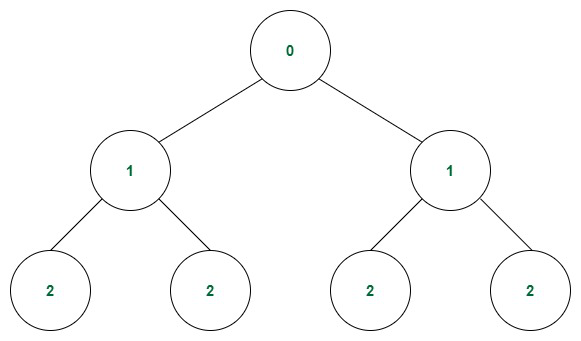# Construct a Perfect Binary Tree with given Height

• Last Updated : 01 Jul, 2022

Given an integer N, the task is to generate a perfect binary tree with height N such that each node has a value that is the same as its depth. Return the inorder traversal of the generated binary tree.

A Perfect binary tree is a type of binary tree where every internal node has exactly two child nodes and all leaf nodes are at same level.

Examples:

Input: N = 2
Output: 2 1 2 0 2 1 2
Explanation: The structure of the tree is as followingPerfect Binary Tree with height 2

Input: N = 1
Output: 1 0 1

Approach: The problem can be solved using level order traversal based on the following observation:

As there is a requirement to fill each node with the value that is the same as its depth so use the level order traversal. In each step fill a total level and mark all the nodes with the value same as the depth.

Follow the steps mentioned below to implement the approach:

• Initiate a variable to store the depth of the current level.
• Initiate a queue to store the node of each level and perform the level order traversal.
• In each iteration get all the nodes in that level and continue the following until the depth is N:
• Increase the depth by 1.
• Pop the nodes at the current level.
• Generate the child nodes for each node.
• Add the new child nodes for further processing.
• After the traversal is complete, the tree is prepared.
• Print the inorder traversal of the tree.

Below is the implementation of the above approach.

## Java

 `// Java code to implement the approach` `import` `java.util.*;` `// Class to hold tree node data``// and left, right children``class` `TreeNode {``    ``public` `long` `val;``    ``public` `TreeNode left;``    ``public` `TreeNode right;` `    ``public` `TreeNode(``long` `x)``    ``{``        ``val = x;``        ``left = ``null``;``        ``right = ``null``;``    ``}``}` `// Class to create the perfect binary tree``class` `BinaryTree {` `    ``// Method to construct a binary tree``    ``public` `static` `TreeNode perfectBinaryTree(``int` `depth)``    ``{``        ``// Return root with 0 as val if height is 0``        ``if` `(depth == ``0``)``            ``return` `new` `TreeNode(``0``);` `        ``// Initiate queue to store``        ``// the nodes on each level``        ``Queue queue``            ``= ``new` `LinkedList<>();` `        ``// Create a root node with value 0``        ``long` `i = ``0``;``        ``TreeNode root = ``new` `TreeNode(i);` `        ``// Add the root node to the queue``        ``// for futher processing``        ``queue.add(root);` `        ``// Iterate through the queue till its empty``        ``while` `(!queue.isEmpty()) {` `            ``// Check the size of the queue to iterate``            ``// through each node on the same level``            ``int` `size = queue.size();` `            ``// Increment the value of node``            ``// upon each level``            ``i++;` `            ``// Break the loop if the value of the node``            ``// reaches given depth``            ``// else process the node in the queue``            ``if` `(i > depth) {``                ``break``;``            ``}``            ``else` `{``                ``// Add the left and right child``                ``// for the node in the queue and``                ``// add those newly created child nodes``                ``// to the queue.``                ``for` `(``int` `j = ``0``; j < size; j++) {``                    ``TreeNode node = queue.remove();``                    ``node.left = ``new` `TreeNode(i);``                    ``node.right = ``new` `TreeNode(i);` `                    ``queue.add(node.left);``                    ``queue.add(node.right);``                ``}``            ``}``        ``}` `        ``// Return the root of the tree``        ``return` `root;``    ``}` `    ``// Inorder traversal of the tree (Left Root Right)``    ``public` `static` `void` `inOrderTraversal(TreeNode node)``    ``{``        ``if` `(node == ``null``)``            ``return``;``        ``inOrderTraversal(node.left);``        ``System.out.print(node.val + ``" "``);``        ``inOrderTraversal(node.right);``    ``}` `    ``// Driver code``    ``public` `static` `void` `main(String[] args)``    ``{``        ``int` `N = ``2``;` `        ``// Function call to build the tree``        ``TreeNode binaryTreeRoot``            ``= perfectBinaryTree(N);` `        ``// Function call to print the tree``        ``inOrderTraversal(binaryTreeRoot);``    ``}``}`

## C#

 `// C# code to implement the approach``using` `System;``using` `System.Collections.Generic;` `// Class to hold tree node data``// and left, right children``public` `class` `TreeNode {``  ``public` `long` `val;``  ``public` `TreeNode left;``  ``public` `TreeNode right;` `  ``public` `TreeNode(``long` `x) {``    ``val = x;``    ``left = ``null``;``    ``right = ``null``;``  ``}``}` `// Class to create the perfect binary tree``public` `class` `BinaryTree {` `  ``// Method to construct a binary tree``  ``public` `static` `TreeNode perfectBinaryTree(``int` `depth) {``    ``// Return root with 0 as val if height is 0``    ``if` `(depth == 0)``      ``return` `new` `TreeNode(0);` `    ``// Initiate queue to store``    ``// the nodes on each level``    ``Queue queue = ``new` `Queue();` `    ``// Create a root node with value 0``    ``long` `i = 0;``    ``TreeNode root = ``new` `TreeNode(i);` `    ``// Add the root node to the queue``    ``// for futher processing``    ``queue.Enqueue(root);` `    ``// Iterate through the queue till its empty``    ``while` `(queue.Count!=0) {` `      ``// Check the size of the queue to iterate``      ``// through each node on the same level``      ``int` `size = queue.Count;` `      ``// Increment the value of node``      ``// upon each level``      ``i++;` `      ``// Break the loop if the value of the node``      ``// reaches given depth``      ``// else process the node in the queue``      ``if` `(i > depth) {``        ``break``;``      ``} ``else` `{``        ``// Add the left and right child``        ``// for the node in the queue and``        ``// add those newly created child nodes``        ``// to the queue.``        ``for` `(``int` `j = 0; j < size; j++) {``          ``TreeNode node = queue.Dequeue();``          ``node.left = ``new` `TreeNode(i);``          ``node.right = ``new` `TreeNode(i);` `          ``queue.Enqueue(node.left);``          ``queue.Enqueue(node.right);``        ``}``      ``}``    ``}` `    ``// Return the root of the tree``    ``return` `root;``  ``}` `  ``// Inorder traversal of the tree (Left Root Right)``  ``public` `static` `void` `inOrderTraversal(TreeNode node) {``    ``if` `(node == ``null``)``      ``return``;``    ``inOrderTraversal(node.left);``    ``Console.Write(node.val + ``" "``);``    ``inOrderTraversal(node.right);``  ``}` `  ``// Driver code``  ``public` `static` `void` `Main(String[] args) {``    ``int` `N = 2;` `    ``// Function call to build the tree``    ``TreeNode binaryTreeRoot = perfectBinaryTree(N);` `    ``// Function call to print the tree``    ``inOrderTraversal(binaryTreeRoot);``  ``}``}` `// This code is contributed by shikhasingrajput`

Output

`2 1 2 0 2 1 2 `

Time Complexity: O(2N)
Auxiliary Space: O(2N)

My Personal Notes arrow_drop_up